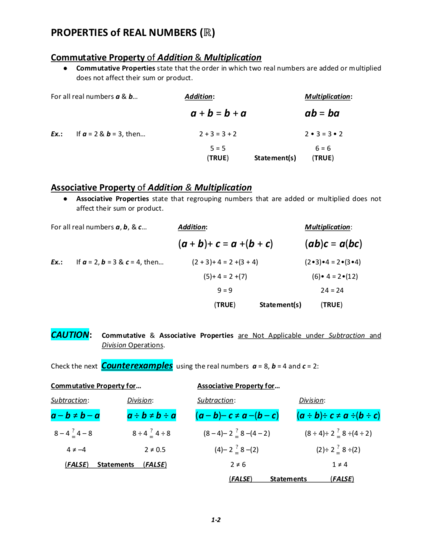# Addition and Multiplication Properties for Real NumbersContributed by:This pdf include:-
Commutative Property
Associative Property
Distributive Property of Multiplication over Addition
Identity Property of Addition & Multiplication
Inverse Property of Addition & Multiplication
1. PROPERTIES of REAL NUMBERS (ℝ)
Commutative Property of Addition & Multiplication
• Commutative Properties state that the order in which two real numbers are added or multiplied
does not affect their sum or product.
For all real numbers a & b… Addition: Multiplication:
a+b=b+a ab = ba
Ex.: If a = 2 & b = 3, then… 2+3=3+2 2•3=3•2
5=5 6=6
(TRUE) Statement(s) (TRUE)
Associative Property of Addition & Multiplication
• Associative Properties state that regrouping numbers that are added or multiplied does not
affect their sum or product.
For all real numbers a, b, & c… Addition: Multiplication:
(a + b)+ c = a +(b + c) (ab)c = a(bc)
Ex.: If a = 2, b = 3 & c = 4, then… (2 + 3)+ 4 = 2 +(3 + 4) (2•3)•4 = 2•(3•4)
(5)+ 4 = 2 +(7) (6)• 4 = 2•(12)
9=9 24 = 24
(TRUE) Statement(s) (TRUE)
CAUTION: Commutative & Associative Properties are Not Applicable under Subtraction and
Division Operations.
Check the next Counterexamples using the real numbers a = 8, b = 4 and c = 2:
Commutative Property for… Associative Property for…
Subtraction: Division: Subtraction: Division:
a–b≠b–a a÷b≠b÷a (a – b)– c ≠ a –(b – c) (a ÷ b)÷ c ≠ a ÷(b ÷ c)
8 – 4 =? 4 – 8 8 ÷ 4 =? 4 ÷ 8 (8 – 4)– 2 =? 8 –(4 – 2) (8 ÷ 4)÷ 2 =? 8 ÷(4 ÷ 2)
4 ≠ –4 2 ≠ 0.5 (4)– 2 =? 8 –(2) (2)÷ 2 =? 8 ÷(2)
(FALSE) Statements (FALSE) 2≠6 1≠4
(FALSE) Statements (FALSE)
1-2
2. PROPERTIES of REAL NUMBERS (ℝ)
Distributive Property of Multiplication over Addition
• Distributive Property states that multiplication distributes over addition or difference between
two or more terms.
For all real numbers a, b, and c, distribute or multiply factor a, as a common factor, over the sum or
difference of the terms b & c…
Multiplication over Addition: Multiplication over Difference:
a ( b + c ) = ab + ac a ( b – c ) = ab – ac
Remember that Condition on Subtraction:
( b – c ) ≠ ( c – b ).
Ex.: Ifa = 2, b = 3, & c = 4, then…
LHS RHS LHS RHS
By Adding the like terms By distributing the factor 2 over By subtracting the like By distributing the factor 2 over the
inside parentheses. the sum inside parentheses. terms inside parentheses. difference inside parentheses.
2(3 + 4) = 2( 3 ) + 2( 4 ) 2( 3 – 4 ) = 2( 3 ) – 2( 4 )
2( 7 ) = 6 + 8 2( – 1 ) = 6 – 8
14 = 14 –2 = –2
(TRUE) Statement(s) (TRUE)
Identity Property of Addition & Multiplication
• Identity Properties state that when adding or multiplying a real number, the result is that same
real number. For all real numbers a…
In Addition, the Additive Identity is ZERO: In Multiplication, the Multiplicative Identity is ONE:
a+0=0+a=a a•1=1•a=a
Inverse Property of Addition & Multiplication
• Inverse Properties state that when adding or multiplying a real number, the result is equal to
such Identity Number, ZERO for Addition and ONE for Multiplication. For all real numbers a,
except 0 for multiplication…
In Addition, the Additive Inverse or Opposite of a is ( –a): a + ( –a) = ( –a) + a = 0 .
𝟏 𝟏 𝟏
In Multiplication, the Multiplicative Inverse or Reciprocal of a is , and a≠0: a• = •a=1.
𝒂 𝒂 𝒂
2-2